#### NCERT Solutions for Class 8th: Ch 6 Squares and Square Roots Maths Part-2

Exercise 6.4

1.Find the square root of each of the following numbers by Division method.
(i) 2304
(ii) 4489
(iii) 3481
(iv) 529
(v) 3249
(vi) 1369
(vii) 5776
(viii) 7921
(ix) 576
(x) 1024
(xi) 3136
(xii) 900

Solution

(i)∴ √2304 = 48

(ii)∴ √4489 = 67

(iii)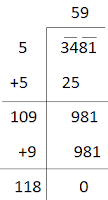∴ √3481 = 59

(iv)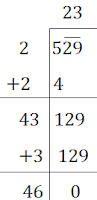∴ √529 = 29

(v)∴  √3249 = 57

(vi)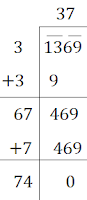∴ √1369 = 37

(vii)∴ √5776 = 76

(viii)∴ √7921 = 89

(ix)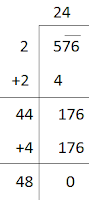∴ √576 = 24

(x)∴ √1024 = 32

(xi)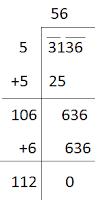∴  √3136 = 56

(xii)∴ √900 = 30

2. Find the number of digits in the square root of each of the following numbers (without any calculation).
(i) 64
(ii) 144
(iii) 4489
(iv) 27225
(v) 390625

Solution

(i)∴  √64 = 8
Hence, the square root of the number 64 has 1 digit.

(ii)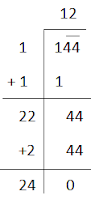∴  √144 = 12
Hence, the square root of the number 144 has 2 digits.

(iii)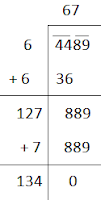∴ √4489 = 67
Hence, the square root of the number 4489 has 2 digits.

(iv)
∴ √27225 = 165
Hence, the square root of the number 27225 has 3 digits.

(v)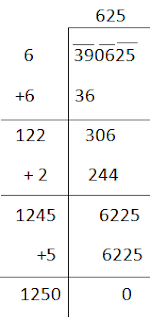∴  √390625 = 625
Hence, the square root of the number 390625 has 3 digits.

3. Find the square root of the following decimal numbers.
(i) 2.56
(ii) 7.29
(iii) 51.84
(iv) 42.25
(v) 31.36

Solution

(i)∴ √2.56 = 1.6

(ii)∴ √7.29 = 2.7

(iii)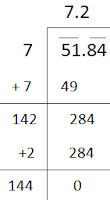∴ √51.84 = 7.2

(iv)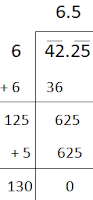∴ √42.25 = 6.5

(v)∴ √31.36 = 5.6

4. Find the least number which must be subtracted from each of the following numbers so as to get a perfect square. Also find the square root of the perfect square so obtained.
(i) 402
(ii) 1989
(iii) 3250
(iv) 825
(v) 4000

Sol: (i)∴ We must subtracted 2 from 402 to get a perfect square.
New number = 402 – 2 = 400∴ √400 = 20

(ii)∴ We must subtracted 53 from 1989 to get a perfect square.
New number = 1989 – 53 = 1936∴ √1936 = 44

(iii)∴ We must subtracted 1 from 3250 to get a perfect square.
New number = 3250 – 1 = 3249∴ √3249 = 57

(iv)∴ We must subtracted 41 from 825 to get a perfect square.
New number = 825 – 41 = 784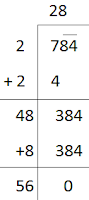∴ √784 = 28

(v)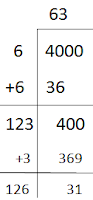∴ We must subtracted 31 from 4000 to get a perfect square.
New number = 4000 – 31 = 3961∴ √3136 = 56

5. Find the least number which must be added to each of the following numbers so as to get a perfect square. Also find the square root of the perfect square so obtained.
(i) 525
(ii) 1750
(iii) 252
(iv) 1825
(v) 6412

Solution

(i)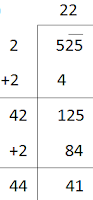Here, (22) < 525 > (23)2
We can say 525 is ( 129 – 125 ) 4 less than (23)2.
∴ If we add 4 to 525, it will be perfect square.
New number = 525 + 4 = 529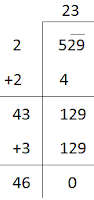∴ √529 = 23

(ii)Here, (41)2 < 1750 > (42)2
We can say 1750 is ( 164 – 150 ) 14 less than (42)2.
∴ If we add 14 to 1750, it will be perfect square.
New number = 1750 + 14 = 1764∴√1764 = 42

(iii)Here, (15) < 252 > (16)2
We can say 252 is ( 156 – 152 ) 4 less than (16)2.
∴ If we add 4 to 252, it will be perfect square.
New number = 252 + 4 = 256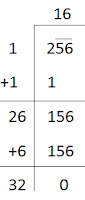∴ √256 = 16

(iv)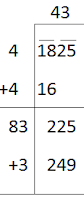Here, (42) < 1825 > (43)2
We can say 1825 is ( 249 – 225 ) 24 less than (43)2.
∴ If we add 24 to 1825, it will be perfect square.
New number = 1825 + 24 = 1849∴ √1849 = 43

(v)Here, (80) < 6412 > (81)2
We can say 6412 is ( 161 – 12 ) 149 less than (81)2.
∴ If we add 149 to 6412, it will be perfect square.
New number = 6412 + 149 = 6561∴ √6561 = 81

6. Find the length of the side of a square whose area is 441 m2.

Solution

Let the length of each side of the field = a
Then, area of the field = 441 m2
⇒ a2 = 441  m2
⇒a = √441 m∴ The length of each side of the field = a m = 21 m.

7. In a right triangle ABC, ∠B = 90°.
(a) If AB = 6 cm, BC = 8 cm, find AC
(b) If AC = 13 cm, BC = 5 cm, find AB

Solution

(a)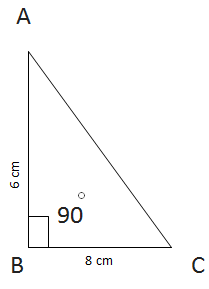Given, AB = 6 cm,
BC = 8 cm
Let AC be x cm.
∴ AC2 = AB2 + BC2Hence, AC = 10 cm.

(b)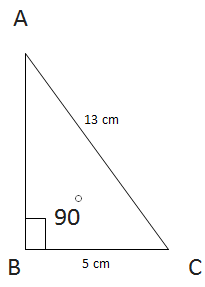Given, AC = 13 cm,
BC = 5 cm
Let AB be x cm.
∴ AC= AB+ BC2
⇒ AC- BC= AB2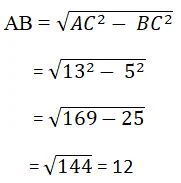Hence, AB = 12 cm

8. A gardener has 1000 plants. He wants to plant these in such a way that the number of rows and the number of columns remain same. Find the minimum number of plants he needs more for this.

Solution

Let the number of rows and column be, x.
∴ Total number of row and column= x×x = x2
As per question, x2 = 1000
⇒ x = √1000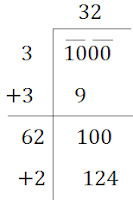Here, (31)2 < 1000 > (32)2
We can say 1000 is ( 124 – 100 ) 24 less than (32)2.
∴ 24 more plants is needed.

9. There are 500 children in a school. For a P.T. drill they have to stand in such a manner that the number of rows is equal to number of columns. How many children would be left out in this arrangement.

Solution

Let the number of rows and column be, x.
∴ Total number of row and column= x × x = x2
As per question, x2 = 500
x = √500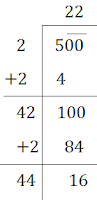Hence, 16 children would be left out in the arrangement.